SSC CGL Previous Year Questions: Geometry- 6

# SSC CGL Previous Year Questions: Geometry- 6 - SSC CGL

Test Description

## 38 Questions MCQ Test SSC CGL (Tier - 1) - Previous Year Papers (Topic Wise) - SSC CGL Previous Year Questions: Geometry- 6

SSC CGL Previous Year Questions: Geometry- 6 for SSC CGL 2023 is part of SSC CGL (Tier - 1) - Previous Year Papers (Topic Wise) preparation. The SSC CGL Previous Year Questions: Geometry- 6 questions and answers have been prepared according to the SSC CGL exam syllabus.The SSC CGL Previous Year Questions: Geometry- 6 MCQs are made for SSC CGL 2023 Exam. Find important definitions, questions, notes, meanings, examples, exercises, MCQs and online tests for SSC CGL Previous Year Questions: Geometry- 6 below.
Solutions of SSC CGL Previous Year Questions: Geometry- 6 questions in English are available as part of our SSC CGL (Tier - 1) - Previous Year Papers (Topic Wise) for SSC CGL & SSC CGL Previous Year Questions: Geometry- 6 solutions in Hindi for SSC CGL (Tier - 1) - Previous Year Papers (Topic Wise) course. Download more important topics, notes, lectures and mock test series for SSC CGL Exam by signing up for free. Attempt SSC CGL Previous Year Questions: Geometry- 6 | 38 questions in 38 minutes | Mock test for SSC CGL preparation | Free important questions MCQ to study SSC CGL (Tier - 1) - Previous Year Papers (Topic Wise) for SSC CGL Exam | Download free PDF with solutions
 1 Crore+ students have signed up on EduRev. Have you?
SSC CGL Previous Year Questions: Geometry- 6 - Question 1

### PQ is a direct common tangent of two circles of radii r1 and r2 touching each other externally at A. Then the value of PQ2 is   (SSC CGL 1st Sit. 2012)

Detailed Solution for SSC CGL Previous Year Questions: Geometry- 6 - Question 1

PQ2 = (r1 + r2)2 – (r1 – r2)2 = 4r1r2

SSC CGL Previous Year Questions: Geometry- 6 - Question 2

### AC and BC are two equal chords of a circle. BA is produced to any point P and CP, when joined cuts the circle at T. Then   (SSC CGL 1st Sit. 2012)

Detailed Solution for SSC CGL Previous Year Questions: Geometry- 6 - Question 2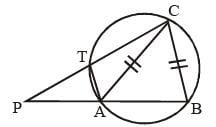In ΔPAC and ΔATC,
∠ATC = ∠PAC = 180° – θ.
∠PAC = ∠TCA
∴ ∠PAC ~ ΔATC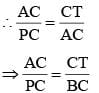⇒ CT : CB = AC : PC

SSC CGL Previous Year Questions: Geometry- 6 - Question 3

### If S is the circumcentre of ΔABC and ∠A = 50°, then the value of ∠BCS is   (SSC CGL 1st Sit. 2012)

Detailed Solution for SSC CGL Previous Year Questions: Geometry- 6 - Question 3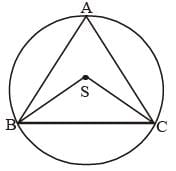∠BAC = 50°
∴ ∠BSC = 100°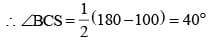SSC CGL Previous Year Questions: Geometry- 6 - Question 4

Let BE and CF the two median s of a ΔABC and G be their intersection. Also let EF cut AG at O. Then AO: OG is   (SSC CGL 1st Sit. 2012)

Detailed Solution for SSC CGL Previous Year Questions: Geometry- 6 - Question 4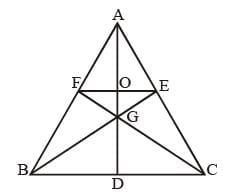Here we extend AG that meet BC at point D.
E and F are mid point, hence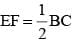As, ΔAFO ~ ΔABD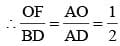∴ O be the mid point of AD i.e. AO = OD.
Now, as centroid divides the median in the ratio 2 : 1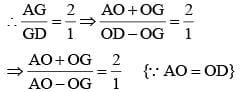2(AO) – 2(OG) = AO + OG.
(AO) = 3(OG)
∴ AO : OG = 3 : 1

SSC CGL Previous Year Questions: Geometry- 6 - Question 5

If the ratio of areas of two similar triangles is 9 : 16, then the ratio of their corresponding sides is    (SSC CGL 1st Sit. 2012)

Detailed Solution for SSC CGL Previous Year Questions: Geometry- 6 - Question 5

Ratio of corresponding sides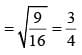SSC CGL Previous Year Questions: Geometry- 6 - Question 6

In an obtuse-angled triangle ABC, ∠A is the obtuse angle and O is the orthocenter.  If ∠BOC = 54°, then ∠BAC is    (SSC CGL 1st Sit. 2012)

Detailed Solution for SSC CGL Previous Year Questions: Geometry- 6 - Question 6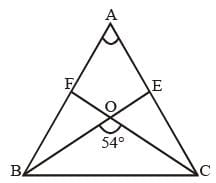Let altitudes drawn from vertex B and C cross each other at Point ‘O’.
then, ∠BOC = 54° = ∠EOF {vertically opposite angles}
∠AEO + ∠AFO + ∠EAF + ∠EOF = 360°
90° + 90° + ∠EAF + 54° = 360°
∴ ∠EAF = 360° – 90° – 90° – 54° = 126°
∴ ∠EAF = ∠BAC = 126°

SSC CGL Previous Year Questions: Geometry- 6 - Question 7

The perimeters of two similar triangle ΔABC and ΔPQR are 36 cm and 24 cm respectively. If PQ = 10 cm, then AB is:   (SSC CGL 2nd Sit. 2012)

Detailed Solution for SSC CGL Previous Year Questions: Geometry- 6 - Question 7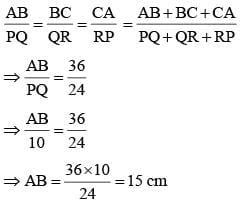SSC CGL Previous Year Questions: Geometry- 6 - Question 8

P is a point outside a circle and is 13 cm away from its centre. A secant drawn from the point P intersect the circle at points A and B in such a way that PA = 9 cm and AB = 7 cm. The radius of the circle is:    (SSC CGL 2nd Sit. 2012)

Detailed Solution for SSC CGL Previous Year Questions: Geometry- 6 - Question 8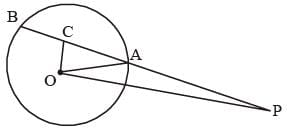OC ⊥ AB
AC = BC = 3.5 cm OP = 13 cm
PC = 9 + 3.5 = 12.5 cm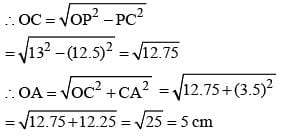SSC CGL Previous Year Questions: Geometry- 6 - Question 9

R and r are the radius of two circles (R > r). If the distance between the centre of the two circles be d, then length of common tangent of two circles is:    (SSC CGL 2nd Sit. 2012)

Detailed Solution for SSC CGL Previous Year Questions: Geometry- 6 - Question 9

Length of common tangent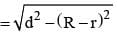SSC CGL Previous Year Questions: Geometry- 6 - Question 10

AB is a diameter of a circle with centre O. CD is a chord equal to the radius of the circle. AC and BD are produced to meet at P. Then the measure of ∠APB is:    (SSC CGL 2nd Sit. 2012)

Detailed Solution for SSC CGL Previous Year Questions: Geometry- 6 - Question 10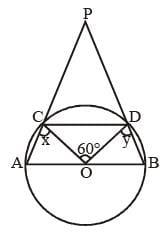Here, in this circle, AO = OB = CO = OD = radius
From question,
CD = CO = OD
∴ ΔOCD is an equilateral triangle.
∴ ∠OCD = ∠ODC = ∠COD = 60°
Now, consider the cyclic quadrileteral ABCD,
∠CAB + ∠BDC = ∠ACD + ∠ABC = 180° ...(i)
Let ∠ACO = x and ∠BDO = y.
then, in ∠AOC, CO = AO = radius
∴ ∠OAC = ∠ACO = x
Similarly in ΔBOD, ∠ODB = ∠BDO = y
Putting these values in equation (i)
∠CAB + ∠BDC = 180°
∠CAB + ∠BDO + ∠ODC = 180°
x + y + 60° = 180°
∴ x + y = 120° ...(ii)
Now, in ΔCPD,
∠PCD = 180° – ∠ACD = 180° – (x + 60°)
and ∠PDC = 180° – ∠BDC = 180° – (y + 60°)
Sum of angles in ΔCPD = 180°
∴ ∠PCD + ∠CPD + ∠PDC = 180°
180° – (x + 60°) + ∠CPD + 180° – (y + 60°) = 180°
60° – (x + y) + ∠CPD = 0
60° – 120° + ∠CPD = 0
∴ ∠CPD = 60°
Hence, ∠APB = 60°

SSC CGL Previous Year Questions: Geometry- 6 - Question 11

The length of the common chord of two circles of radii 15 cm and 20 cm whose centres are 25 cm apart is (in cm):    (SSC CGL 2nd Sit. 2012)

Detailed Solution for SSC CGL Previous Year Questions: Geometry- 6 - Question 11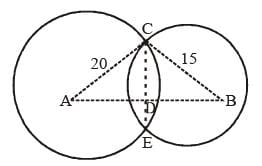Let two circles with centre A and B intersect each other such at points C and E such that CE is its common chord.
As A and B are the centre of the circles So, line AB divides chord CE at point D in two equal parts such that CD = DE and also, AB ⊥ CE
Now, Consider ΔACD and ΔBCD,
AC = 20 cm, BC = 15 cm (given)
Let, CD = x cm
and AD = y cm then BD = (25 – y) cm.
(20)2 = y2 + x2 ...(i)
From ΔBDC, (BC)2 = (BD)2 + (CD)2
(15)2 = (25 – y)2 + x2 ...(ii)
From equation (i) and (ii), we have
(20)2 – (15)2 = y2 – (25 – y)2
(20 + 15) (20 – 15) = (y – 25 + y) (y + 25 – y)
35 × 5 = 25 (2y – 25)
2y = 7 + 25 = 32
y = 16
Again, from equation (i),
(20)2 = (16)2 + x2
∴ x2 = (20)2 – (16)2
= 400 – 256 = 144
CD = x = 12 cm.
Common chord CE = 2 × CD = 2 × 12 = 24 cm

SSC CGL Previous Year Questions: Geometry- 6 - Question 12

D and E are the mid-points of AB and AC of DABC; BC is produced to any point P; DE, DP and EP are joined. Then,    (SSC CGL 2nd Sit. 2012)

Detailed Solution for SSC CGL Previous Year Questions: Geometry- 6 - Question 12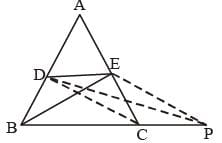In ΔABC, point D and E are mid point of side AB and AC.
So, CD is the median of ΔABC.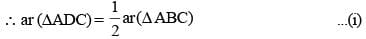Again, from ΔACD, ED is median of ΔACD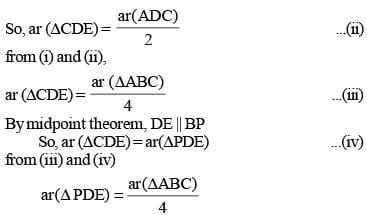SSC CGL Previous Year Questions: Geometry- 6 - Question 13

O is the circumcentre of the triangle ABC with circumradius 13 cm. Let BC = 24 cm and OD is perpendicular to BC. Then the length of OD is:    (SSC CGL 2nd Sit. 2012)

Detailed Solution for SSC CGL Previous Year Questions: Geometry- 6 - Question 13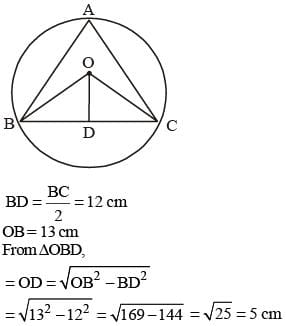SSC CGL Previous Year Questions: Geometry- 6 - Question 14

By decreasing 15° of each an gle of a trian gle, the ratios of their angles are 2 : 3 : 5. The radian measure of greatest angle is:    (SSC CGL 2nd Sit. 2012)

Detailed Solution for SSC CGL Previous Year Questions: Geometry- 6 - Question 14

2x + 3x + 5x = 180°  –  45°  = 135°
⇒ 10x = 135°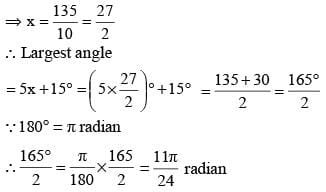SSC CGL Previous Year Questions: Geometry- 6 - Question 15

If G is the centroid of ΔABC and AG = BC, then ∠BGC is:   (SSC CGL 2nd Sit. 2012)

Detailed Solution for SSC CGL Previous Year Questions: Geometry- 6 - Question 15In ΔABC
Given AG = BC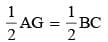i.e., GD = BD = DC
In ΔBGD
BD = DG ∴ ∠GBD = ∠DGB …(i)
In ΔCGD
GD = DC, ∴ ∠GCD = ∠DGC …(ii)
∠GBD + ∠DGB + ∠DGC + ∠DCG = 180
2(∠BGD + ∠CGD) = 180

SSC CGL Previous Year Questions: Geometry- 6 - Question 16

ΔABC and ΔDEF are similar and their areas be respectively 64 cm2 and 121 cm2. If EF = 15.4 cm, BC is:   (SSC CGL 2nd Sit. 2012)

Detailed Solution for SSC CGL Previous Year Questions: Geometry- 6 - Question 16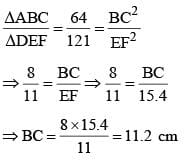SSC CGL Previous Year Questions: Geometry- 6 - Question 17

The perimeter of an isosceles, right-angled triangle is 2p unit. The area of the same triangle is:   (SSC CGL 2nd Sit. 2012)

Detailed Solution for SSC CGL Previous Year Questions: Geometry- 6 - Question 17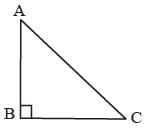AB = BC = x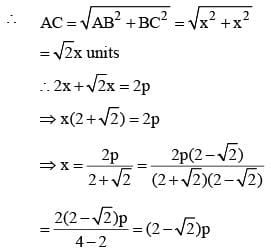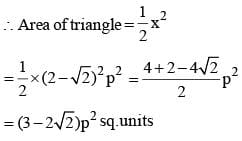SSC CGL Previous Year Questions: Geometry- 6 - Question 18

In ΔABC, AD is the median and AD = 1/2 BC. If ∠BAD = 30°, then measure of ∠ACB is   (SSC CGL 1st Sit. 2012)

Detailed Solution for SSC CGL Previous Year Questions: Geometry- 6 - Question 18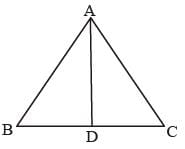In ΔABD,
∴ ∠ABD = ∠BAD = 30°
∴ ∠ADB = 180° – 2 × 30° = 120°
∴ ∠ADC = 180° – 120° = 60°
⇒ ∠DAC = ∠ACD = 60°

SSC CGL Previous Year Questions: Geometry- 6 - Question 19

Two sides of a triangle are of length 4 cm and 10 cm. If the length of the third side is 'a' cm. then   (SSC CGL 1st Sit. 2012)

Detailed Solution for SSC CGL Previous Year Questions: Geometry- 6 - Question 19

The sum of any two sides of a triangle is greater than third side and their difference is less than third side.
10 – 4 < a < 10 + 4
6 < a < 14

SSC CGL Previous Year Questions: Geometry- 6 - Question 20

The angle formed by the hour–hand and the minute–hand of a clock at 2 : 15 p.m. is   (SSC CGL 1st Sit. 2012)

Detailed Solution for SSC CGL Previous Year Questions: Geometry- 6 - Question 20

Angle traced by hour hand in an hour = 30°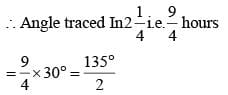Angle traced by minute hand in 60 minutes = 360°
∴ Angle traced in 15 minutes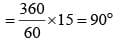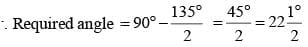SSC CGL Previous Year Questions: Geometry- 6 - Question 21

If the length of the side PQ of the rhombus PQRS is 6 cm and ∠PQR = 120°, then the length of QS, in cm, is   (SSC CGL 1st Sit. 2012)

Detailed Solution for SSC CGL Previous Year Questions: Geometry- 6 - Question 21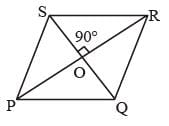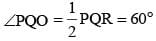From ΔPOQ,
∠OPQ = 180° – 90° – 60° = 30°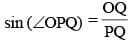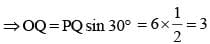∴ QS = 2 × 3 = 6 cm

SSC CGL Previous Year Questions: Geometry- 6 - Question 22

If each interior angle is double of each exterior angle of a regular polygon with n sides, then the value of n is   (SSC CGL 1st Sit. 2012)

Detailed Solution for SSC CGL Previous Year Questions: Geometry- 6 - Question 22

Let exterior angle = x
then, interior ∠be = 2x
x + 2x = 180
3x = 180
x = 60°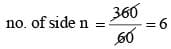SSC CGL Previous Year Questions: Geometry- 6 - Question 23

Two tangents are drawn from a point P to a circle at A and B. O is the centre of the circle. If ∠AOP = 60°, then ∠APB is    (SSC CGL 1st Sit. 2012)

Detailed Solution for SSC CGL Previous Year Questions: Geometry- 6 - Question 23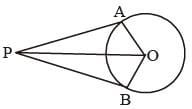In right Δs OAP and OPB,
AP = PB, OA = OB
OP = OP
∴ ΔOAP ≌ ΔOBP
∴ ∠AOP = ∠POB and ∠APO = ∠OPB
From ΔAOP,
∠APO = 180° – 90° – 60° = 30°
∴ ∠APB = 2 × 30 = 60°

SSC CGL Previous Year Questions: Geometry- 6 - Question 24

Two circles of radii 4 cm and 9 cm respectively touch each other externally at a point and a common tangent touches them at the points P and Q respectively. They the area of a square with one side PQ, is   (SSC CGL 1st Sit. 2012)

Detailed Solution for SSC CGL Previous Year Questions: Geometry- 6 - Question 24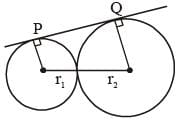r1 + r2 = 13 cm
r2 – r1 = 9 – 4 = 5 cm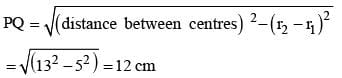∴ Area of square of side PQ = 12 × 12 = 144 sq. cm.

SSC CGL Previous Year Questions: Geometry- 6 - Question 25

The side BC of ΔABC is produced to D. If ∠ACD = 108° and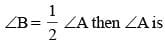(SSC CGL 1st Sit. 2012)

Detailed Solution for SSC CGL Previous Year Questions: Geometry- 6 - Question 25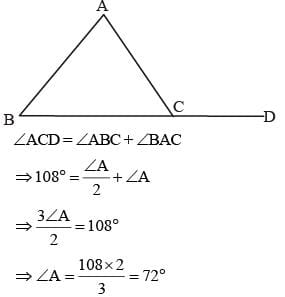SSC CGL Previous Year Questions: Geometry- 6 - Question 26

In the following figure, O is the centre of the circle and XO is perpendicular to OY. If the area of the triangle XOY  is 32, then the area of the circle is   (SSC CGL 1st Sit. 2012)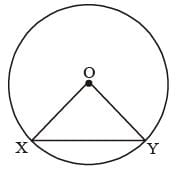Detailed Solution for SSC CGL Previous Year Questions: Geometry- 6 - Question 26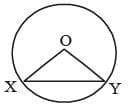∠XOY = 90°; OX = OY = radices (r)
∴ ΔXOY is a right angled triangle.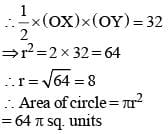SSC CGL Previous Year Questions: Geometry- 6 - Question 27

A, O, B are three points on a line segment and C is a point not lying on AOB. If ∠AOC = 40° and OX, OY are the internal and external bisectors of ∠AOC respectively, then ∠BOY is    (SSC CGL 1st Sit. 2012)

Detailed Solution for SSC CGL Previous Year Questions: Geometry- 6 - Question 27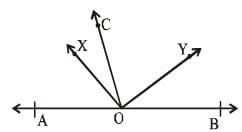OX is the bisector of ∠AOC.
∴ ∠AOC = 2 ∠COX
OY is the bisector of ∠BOC.
∴ ∠BOC = 2 ∠COY
∴ ∠AOC + ∠BOC
= 2∠COY + 2∠COX = 180°
⇒ 2 (∠COX + ∠YOC) = 180°
⇒ ∠XOY = 90°
∴ ∠AOX + ∠XOY + ∠BOY = 180°
∴ ∠BOY = 180° – 90° – 20° = 70°

SSC CGL Previous Year Questions: Geometry- 6 - Question 28

The length of radius of a circumcircle of a triangle having sides 3 cm, 4 cm and 5 cm is:    (SSC CHSL 2012)

Detailed Solution for SSC CGL Previous Year Questions: Geometry- 6 - Question 28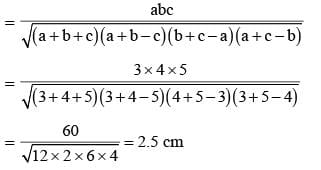SSC CGL Previous Year Questions: Geometry- 6 - Question 29

The length of the two sides forming the right angle of a right-angled triangle are 6 cm and 8 cm. The length of its circumradius is:    (SSC CHSL 2012)

Detailed Solution for SSC CGL Previous Year Questions: Geometry- 6 - Question 29

In a right angled D, the length of circumradius is half the length of hypotenuse.
∴ H2 = 62 + 82
H2 = 36 + 64 ⇒ H2 = 100
H = 10 cm

SSC CGL Previous Year Questions: Geometry- 6 - Question 30

If ΔABC is an isosceles triangle with ∠C = 90º and AC = 5 cm, then AB is:   (SSC CHSL 2012)

Detailed Solution for SSC CGL Previous Year Questions: Geometry- 6 - Question 30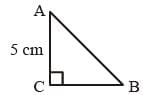ΔABC is an isosceles triangle.
Therefore, AC = BC = 5 cm
Now, AB2 = AC2 + BC2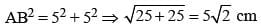SSC CGL Previous Year Questions: Geometry- 6 - Question 31

ABC is a right angled triangle, right angled at C and p is the length of the perpendicular from C on AB. If a, b and c are the lengths of the sides BC, CA and AB respectively, then   (SSC CHSL 2012)

Detailed Solution for SSC CGL Previous Year Questions: Geometry- 6 - Question 31

Here,
∠ACB = 90°
∠BDC = 90°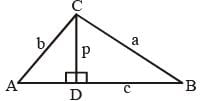Triangles ACB, ADC and BDC are right angle triangles.
Here, Area of ΔABC = Area of ΔADC + Area of ΔBDC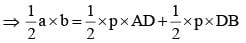⇒ ab = p (AD + DB)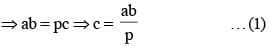Now, In ΔABC,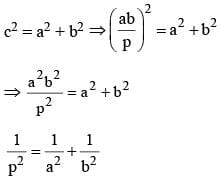SSC CGL Previous Year Questions: Geometry- 6 - Question 32

If in ΔABC, ∠A = 90°, BC = a, AC = b and AB = c, then the value of tan B + tan C is    (SSC Sub. Ins. 2012)

Detailed Solution for SSC CGL Previous Year Questions: Geometry- 6 - Question 32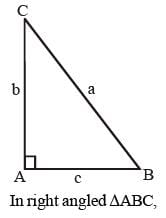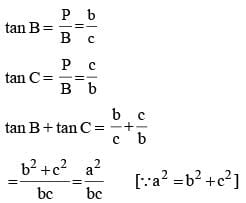SSC CGL Previous Year Questions: Geometry- 6 - Question 33

In the following figure, if OA = 10 and AC = 16, then OB must be   (SSC Sub. Ins. 2012)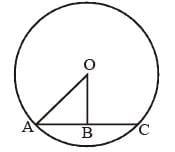Detailed Solution for SSC CGL Previous Year Questions: Geometry- 6 - Question 33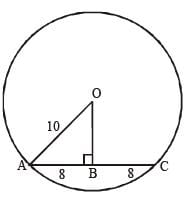In OAB,
OA2 = OB2 + AB2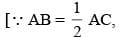because line drawn from centre to a chord bisect & perpendicular to it]
(10)2 = (OB)2 + (8)2
100 – 64 = OB2
OB2 = 36
OB = 6

SSC CGL Previous Year Questions: Geometry- 6 - Question 34

G is the centroid of ΔABC. If AG = BC, then ∠BGC is     (SSC Sub. Ins. 2012)

Detailed Solution for SSC CGL Previous Year Questions: Geometry- 6 - Question 34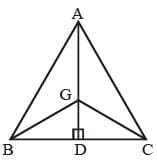AG = BC (Given)
BD = DC (given) and AD is median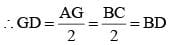So, GD = BD = DC
ΔBGD & ΔGCD are both isosceles D.
Then ∠BGC = ∠BGD + ∠CGD = 90°SSC CGL Previous Year Questions: Geometry- 6 - Question 35

PA and PB are two tangents drawn from an external point P to a circle with centre O where the points A and B are the points of contact. The quadrilateral OAPB must be   (SSC Sub. Ins. 2012)

Detailed Solution for SSC CGL Previous Year Questions: Geometry- 6 - Question 35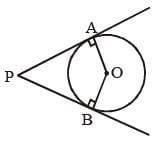OAPB is concyclic because ∠A + ∠B = 180°
& ∠O + ∠P = 180°

SSC CGL Previous Year Questions: Geometry- 6 - Question 36

In the figure below, if AB || CD and CE ⊥ ED, then the value of x is   (SSC Sub. Ins. 2012)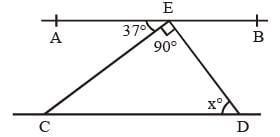Detailed Solution for SSC CGL Previous Year Questions: Geometry- 6 - Question 36

∠AEC = ∠ECD (Alternate interior angles as AB || CD)
In ΔCED,
∠ECD + ∠CED + x° = 180°
(Sum of angles of a triangle are 180°)
37° + 90° + x° = 180°
x° = 180° – 37° – 90°
x° = 53°

SSC CGL Previous Year Questions: Geometry- 6 - Question 37

In ΔABC, ∠B = 60°, ∠C = 40°. If AD bisects ∠BAC and AE ⊥ BC, then ∠EAD is   (SSC Sub. Ins. 2012)

Detailed Solution for SSC CGL Previous Year Questions: Geometry- 6 - Question 37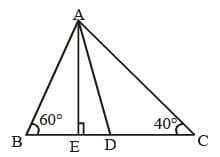In ΔABC,
∠A + ∠B + ∠C = 180°
∠A + 60° + 40° = 180°
∠A = 180° – 60° – 40° = 80°
∴ ∠A = ∠BAD + ∠DAC
Now, In DABE
∠B + ∠E + ∠BAE = 180°
60° + 90° + ∠BAE = 180°
∠BAE = 30°
∴ ∠EAD = ∠BAD – ∠BAE = 40° – 30° = 10°

SSC CGL Previous Year Questions: Geometry- 6 - Question 38

ABCD is a quadrilateral in which diagonal BD = 64 cm, AL ⊥ BD and CM ⊥ BD, such that AL = 13.2 cm and CM = 16.8 cm. The area of the quadrilateral ABCD (in square centimetres) is    (SSC Sub. Ins. 2012)

Detailed Solution for SSC CGL Previous Year Questions: Geometry- 6 - Question 38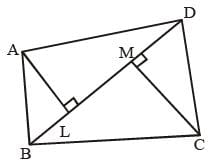Given:
BD = 64 cm
AL = 13.2 cm
CM = 16.8 cm
So, Area (ABCD) = Area (ΔABD) + Area (ΔBCD)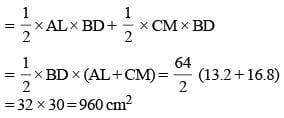## SSC CGL (Tier - 1) - Previous Year Papers (Topic Wise)

250 tests
Information about SSC CGL Previous Year Questions: Geometry- 6 Page
In this test you can find the Exam questions for SSC CGL Previous Year Questions: Geometry- 6 solved & explained in the simplest way possible. Besides giving Questions and answers for SSC CGL Previous Year Questions: Geometry- 6, EduRev gives you an ample number of Online tests for practice

250 tests